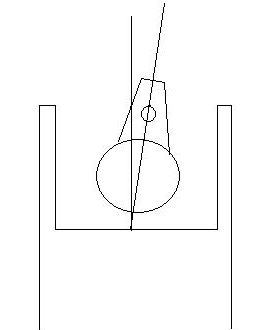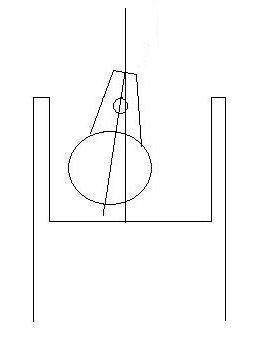# Using higher mathematics in RC

When you were a kid in school, did you ever wonder when you would use trigonometry or algebra?  Maybe math would seem more useful if it could help you build RC airplanes.  I use a little bit of trigonometry and algebra to figure out where to place my engine to get the correct amount of right thrust.

Let’s say you are building a plane and you drill your firewall to place the engine mount right in the center.  Then you notice that the plans call for 3 degrees of right thrust, so you shim the left side of the mount.  Now the center of your propeller is is noticeably offset to the right.  I hate it when that happens.  It just doesn’t look right.I like to calculate how far to one side the propeller shaft is going to land, then move the back of the engine to the left.  That way the propeller will be centered.It’s not a hard calculation, and a scientific calculator makes it even easier.  The trigonometric function of the Sine tells us that the sine of an angle equals the length of the opposite side divided by the hypotenuse (S=O/H).

Here’s a diagram showing the centerline of the airplane as one side of a right triangle, and the centerline of the engine as the hypotenuse.  (For those who don’t remember math lessons, the hypotenuse is the longest side of a right triangle.)Your engine and mount are 4 inches long, from the firewall to the back of the propeller.  X is the distance you will offset your mount to the left.  According to the trigonometry book, the sine of 3 degrees = X/4 inches.  Punch some buttons on your calculator and you’ll find that Sin3 = .0523.

Therefore, .0523 = X/4.  If you remember your algebra lessons, you know that whenever you have an equation with the = sign in the middle, it means that you can add, subtract, multiply or divide any number as long as you do it to both sides.  Multiply both sides of the equation by 4 and you get:

4 times .0523 = X

In other words, the amount of leftward offset  (the length of the little line in the triangle diagram) equals .21 inch, or just a hair less than 1/4″.  So you drill your engine mount holes just about a quarter of an inch to the left of the centerline.  When you shim your mount to get 3 degrees of right thrust, the propeller shaft will land in the middle.

To simplify things even more, here’s an equation using RC terms that will be easy to remember. SinA x L = Offset, where A is the thrust angle and L is engine length.

Let’s say you have a big four stroke with a big propeller being mounted on a biplane.  This type of installation would require considerable offset, so let’s say it’s going to be 5.5 degrees.  This is a 1.20 engine with a total length of 6 inches including mount.  With such a long engine and extreme thrust angle, this plane would look ridiculous if it were mounted at the centerline with the propeller shaft way over on the right side, so let’s get it right from the beginning.  Here’s the equation and the solution:

SinA x L = Offset

Sin5.5 = .096, and L = 6″

.096 x 6″ = .575″

.575 is approximately 9/16″, which is how far you move your engine mount to the left.